GMAT  >  Test: Data Sufficiency- 4

# Test: Data Sufficiency- 4

Test Description

## 10 Questions MCQ Test Quantitative for GMAT | Test: Data Sufficiency- 4

Test: Data Sufficiency- 4 for GMAT 2022 is part of Quantitative for GMAT preparation. The Test: Data Sufficiency- 4 questions and answers have been prepared according to the GMAT exam syllabus.The Test: Data Sufficiency- 4 MCQs are made for GMAT 2022 Exam. Find important definitions, questions, notes, meanings, examples, exercises, MCQs and online tests for Test: Data Sufficiency- 4 below.
Solutions of Test: Data Sufficiency- 4 questions in English are available as part of our Quantitative for GMAT for GMAT & Test: Data Sufficiency- 4 solutions in Hindi for Quantitative for GMAT course. Download more important topics, notes, lectures and mock test series for GMAT Exam by signing up for free. Attempt Test: Data Sufficiency- 4 | 10 questions in 20 minutes | Mock test for GMAT preparation | Free important questions MCQ to study Quantitative for GMAT for GMAT Exam | Download free PDF with solutions
 1 Crore+ students have signed up on EduRev. Have you?
Test: Data Sufficiency- 4 - Question 1

### If w, x, y, and z are the digits of the four-digit number N, a positive integer, what is the remainder when N is divided by 9? 1) w + x + y + z = 13 2) N + 5 is divisible by 9

Detailed Solution for Test: Data Sufficiency- 4 - Question 1

1. In order for a number, n, to be divisible by 9, its digits must add to nine. Likewise, the remainder of the sum of the digits of n divided by 9 is the remainder when n is divided by 9. In other words:

2. To see this, consider a few examples:
Let N = 901
901/9 = 100 + (R = 1)
(9+0+1)/9 = 10/9 = 1 + (R = 1)

Let N = 85
85/9 = 9 + (R = 4)
(8+5)/9 = 1 + (R = 4)

Let N = 66
66/9 = 7 + (R = 3)
(6+6)/9 = 1 + (R = 3)

Let N = 8956
8956/9 = 995 + (R = 1)
(8+9+5+6)/9 = 28/9 = 3 + (R = 1)

3.

Based upon what was shown above, since the sum of the digits of N is always 13, we know that remainder of N/9 will always be the remainder of 13/9, which is R=4.
In case this is hard to believe, consider the following examples:
4540/9 = 504 + (R = 4)
(4+5+4+0)/9 = 13/9 = 1 + (R = 4)

1390/9 = 154 + (R = 4)
(1+3+9+0)/9 = 13/9 = 1 + (R = 4)

7231/9 = 803 + (R = 4)
(7+2+3+1)/9 = 13/9 = 1 + (R = 4)

1192/9 = 132 + (R = 4)
(1+1+9+2)/9 = 13/9 = 1 + (R = 4)
Statement (1) is SUFFICIENT.
4.  Since Statement (1) alone is SUFFICIENT and Statement (2) alone is SUFFICIENT, answer D is correct.

Test: Data Sufficiency- 4 - Question 2

### After a long career, John C. Walden is retiring. If there are 25 associates who contribute equally to a parting gift for John in an amount that is an integer, what is the total value of the parting gift? 1) If four associates were fired for underperformance, the total value of the parting gift would have decreased by \$200 2) The value of the parting gift is greater than \$1,225 and less than \$1,275

Detailed Solution for Test: Data Sufficiency- 4 - Question 2

Simplify the question by translating it into algebra.
Let P = the total value of John's parting gift
Let E = the amount each associate contributed
Let N = the number of associates
P = NE = 25E
With this algebraic equation, if you find the value of either P or E, you will know the total value of the parting gift.
Evaluate Statement (1) alone.
Two common ways to evaluate Statement (1) alone:
Statement 1: Method 1
Since the question stated that each person contributed equally, if losing four associates decreased the total value of the parting gift by \$200, then the value of each associate's contribution was \$50 (=\$200/4).
Consequently, P = 25E = 25(50) = \$1,250.
Statement 1: Method 2
If four associates leave, there are N - 4 = 25 - 4 = 21 associates.
If the value of the parting gift decreases by \$200, its new value will be P - 200.
Taken together, Statement (1) can be translated:
P - 200 = 21E
P = 21E + 200
You now have two unique equations and two variables, which means that Statement (1) is SUFFICIENT.
Although you should not spend time finding the solution on the test, here is the solution.
Equation 1: P = 21E + 200
Equation 2: P = 25E
P = P
25E = 21E + 200
4E = 200
E = \$50
P = NE = 25E = 25(\$50) = \$1250
Evaluate Statement (2) alone.
Statement (2) says that \$1,225 < P < \$1,275. It is crucial to remember that the question stated that "25 associates contribute equally to a parting gift for John in an amount that is an integer." In other words P / 25 must be an integer. Stated differently, P must be a multiple of 25.
There is only one multiple of 25 between 1,225 and 1,275. That number is \$1,250. Since there is only one possible value for P, Statement (2) is SUFFICIENT.
Since Statement (1) alone is SUFFICIENT and Statement (2) alone is SUFFICIENT, answer D is correct.

Test: Data Sufficiency- 4 - Question 3

### If x and y are distinct positive integers, what is the value of x4-y4? 1)(y2+ x2)(y + x)(x -y) = 240 2)xy= yx and x > y

Detailed Solution for Test: Data Sufficiency- 4 - Question 3

Before even evaluating the statements, simplify the question. In a more complicated data sufficiency problem, it is likely that some rearranging of the terms will be necessary in order to see the correct answer.
Use the formula for a difference of squares (a2 - b2) = (a + b)(a - b). However, let x2 equal a, meaning a2 = x4.
x4 - y4 = (x2 + y2)(x2 - y2)
Recognize that the expression contains another difference of squares and can be simplified even further.
(x2 + y2)(x2 – y2) = (x2 + y2)(x – y)(x + y)
The question can now be simplified to: "If x and y are distinct positive integers, what is the value of (x2 + y2)(x – y)(x + y)?" If you can find the value of (x2 + y2)(x - y)(x + y) or x4 - y4, you have sufficient data.
Evaluate Statement (1) alone.
Statement (1) says (y2 + x2)(y + x)(x - y) = 240. The information in Statement (1) matches exactly the simplified question. Statement (1) is SUFFICIENT.
Evaluate Statement (2) alone.
Statement (2) says xy = yx and x > y. In other words, the product of multiplying x together y times equals the product of multiplying y together x times.
The differences in the bases must compensate for the fact that y is being multiplied more times than x (since x > y and y is being multiplied x times while x is being multiplied y times).
4 and 2 are the only numbers that work because only 4 and 2 satisfy the equation n2 = 2n, which is the condition that would be necessary for the equation to hold true.
Observe that this is true: 42 = 24 = 16.
Remember that x > y, so x = 4 and y = 2. Consequently, you know the value of x4 - y4 from Statement (2). So, Statement (2) is SUFFICIENT.
Since Statement (1) alone is SUFFICIENT and Statement (2) alone is SUFFICIENT

Test: Data Sufficiency- 4 - Question 4

If z = xn-19, is z divisible by 9?
1)x = 10; n is a positive integer
2)z + 981 is a multiple of 9

Detailed Solution for Test: Data Sufficiency- 4 - Question 4

In working on this question, it is helpful to remember that a number will be divisible by 9 if the sum of its digits equals 9.
Evaluate Statement (1) alone.
Based upon the information in Statement (1), it is helpful to plug in a few values and see if a pattern emerges:
101 - 19 = -9
102 - 19 = 81; the sum of the digits is 9, which is divisible by 9, meaning the entire expression is divisible by 9
103 - 19 = 981; the sum of the digits is 9 + 8 + 1=18, which is divisible by 9, meaning the entire expression is divisible by 9
104 - 19 = 9981; the sum of the digits is 9(2) + 8 + 1=27, which is divisible by 9, meaning the entire expression is divisible by 9
Notice that, in each instance, the sum of the digits is divisible by 9, meaning the entire expression is divisible by 9.
The pattern that emerges is that there are (n-2) 9s followed by the digit 8 and the digit 1.
The pattern of the sum of the digits of 10n - 19 is 9(n-2) + 9 for all values of n > 1. (For n = 1, the sum is -9, which is also divisible by 9.) This means that the sum of the digits of 10n - 19 is 9(n-1). Since this sum will always be divisible by 9, the entire expression (i.e., 10n - 19) will always be divisible by 9.
Based upon this pattern, Statement (1) is SUFFICIENT.
Evaluate Statement (2) alone.
Statement (2) says that z + 981 is a multiple of 9. This can be translated into algebra: 9(a constant integer) = z + 981
Divide both sides by 9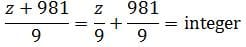Since 981 is divisible by 9 (its digits sum to 18, which is divisible by 9), you can further rewrite Statement (2).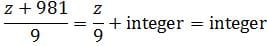Since an integer minus an integer is an integer, Statement (2) can be rewritten even further. Since z divided by 9 is an integer, z is divisible by 9. Statement (2) is SUFFICIENT.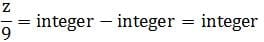Since Statement (1) alone is SUFFICIENT and Statement (2) alone is SUFFICIENT.

Test: Data Sufficiency- 4 - Question 5

x is a positive integer; what is the value of x?
1)The sum of any two positive factors of x is even
2)x is a prime number and x < 4

Detailed Solution for Test: Data Sufficiency- 4 - Question 5

Evaluate Statement (1) alone.
Statement (1) says that the sum of any two factors is even. The sum of two integers is even under two circumstances:
odd + odd = even
even + even = even
Since the sum of any two factors is even, all the factors must have the same parity. If x had both even and odd factors, then it would be possible for two factors to add together and be odd (remember that an odd number + an even number = an odd number and, in Statement 1, the sum of any two positive factors must be even).
Since the problem says "the sum of any two positive factors of x is even" and the number 1 is a factor of any number, x must only contain odd factors. If x contained one even factor, it would be possible to add that even factor with the number one and the result would be an odd number. Since the number 2 is a factor of every even number, x cannot be even. Otherwise, it would be possible to add the factors 1 and 2 together and their sum would not be even.
Statement (1), when inspected carefully, says that x is an odd number that only contains odd factors. Since there are many possibilities (x = 1, 3, 5, 7, 9, 11, 15, ...), Statement (1) is NOT SUFFICIENT.
Evaluate Statement (2) alone.
Statement (2) says that x is a prime number less than 4. Remember that x must also be a positive integer (as per the original question). Although this narrows the possibilities for x, because there are still two possibilities (x = 2 or x = 3; both these values are prime, less than 4, and positive integers), Statement (2) is NOT SUFFICIENT. Please remember that the number one is not prime.
Evaluate Statements (1) and (2) together.
Statements (1) and (2), when taken together, definitively show that x = 3. Statements (1) and (2), when taken together, are SUFFICIENT. Answer choice C is correct.

Test: Data Sufficiency- 4 - Question 6

What is the value of x?
1)The average (arithmetic mean) of 5, x2, 2, 10x, and 3 is -3
2)The medianof 109, -32, -30, 208, -15, x, 10, -43, 7 is -5

Detailed Solution for Test: Data Sufficiency- 4 - Question 6

Evaluate Statement (1) alone.
Based upon the formula for the average, you know that:
(5 + x2 + 2 + 10x + 3)/5 = -3
x2 + 10x + 5 + 2 + 3 = -15
x2 + 10x + 5 + 2 + 3 + 15 = 0
x2 + 10x + 25 = 0
(x + 5)2 = 0
x = -5
Statement (1) alone is SUFFICIENT.
Evaluate Statement (2) alone.
Order the numbers in ascending order without x:
-43, -32, -30, -15, 10, 7, 109, 208
Consider the possible placements for x and whether these would make the median equal to -5:
Case (1): x, -43, -32, -30, -15, 10, 7, 109, 208
Median: -15
Not a possible case since the median is not -5.

Case (2): -43, x, -32, -30, -15, 10, 7, 109, 208
Median: -15
Not a possible case since the median is not -5.

Case (3): -43, -32, x, -30, -15, 10, 7, 109, 208
Median: -15
Not a possible case since the median is not -5.

Case (4): -43, -32, -30, x, -15, 10, 7, 109, 208
Median: -15
Not a possible case since the median is not -5.

Case (5): -43, -32, -30, -15, x, 10, 7, 109, 208
Median: x
A possible case since the median is x, which can legally be -5.
In this case, x must be -5 in order for the median of the set to be -5, which must be according to Statement (2).

Case (6): -43, -32, -30, -15, 10, x, 7, 109, 208
Median: 10
Not a possible case since the median is not -5.

Case (7): -43, -32, -30, -15, 10, 7, x, 109, 208
Median: 10
Not a possible case since the median is not -5.

Case (8): -43, -32, -30, -15, 10, 7, 109, x, 208
Median: 10
Not a possible case since the median is not -5.

Case (9): -43, -32, -30, -15, 10, 7, 109, 208, x
Median: 10
Not a possible case since the median is not -5.
Since Statement (2) tells us that the median must be -5, we know that x must be a value such that the median is -5. This can only happen in Case 5. Specifically, it can only happen when x = -5. Since the median must equal -5 and this can only happen when x = -5, we know that x = -5.
Statement (2) alone is SUFFICIENT.
Since Statement (1) alone is SUFFICIENT and Statement (2) alone is SUFFICIENT

Test: Data Sufficiency- 4 - Question 7

In 2003, a then-nascent Internet search engine developed an indexing algorithm called G-Cache that retrieved and stored X million webpages per hour. At the same time, a competitor developed an indexing algorithm called HTML-Compress that indexed and stored Y million pages per hour. If both algorithms indexed a positive number of pages per hour, was the number of pages indexed per hour by G-Cache greater than three times the number of pages indexed by HTML-Compress?
1)On a per-hour basis in 2003, G-Cache indexed 1 million more pages than HTML-Compress indexed
2)HTML-Compress can index between 400,000 and 1.4 million pages per hour

Detailed Solution for Test: Data Sufficiency- 4 - Question 7

Translate the final sentence, which contains the question, into algebra:
"the number of pages indexed per hour by G-Cache" = X
"greater than three times" translates into: >3
"the number of pages indexed by HTML-Compress" = Y

Putting this together:
Was X > 3Y?
Evaluate Statement (1) alone.
Translate the information from Statement (1) into algebra:
X - Y = 1 million
Since the original question states that "both algorithms indexed a positive number of pages per hour", the following inequalities must hold true:
X > 0
Y > 0
Simply knowing that X - Y = 1 million does not provide enough information to determine whether X > 3Y.
This can be seen via an algebraic substitution or by trying different numbers.
Trying Numbers
Let X = 10 and, therefore, Y = 9
10 is NOT > 3(9)
But, let X = 1.1 and, therefore, Y = .1
1.1 IS > 3(.1)
Algebraic Substitution
X - Y = 1 million
X = Y + 1 million
Plug this into the inequality we are trying to solve for:
Was X > 3Y?
Was (Y + 1 million) > 3Y?
Was 1 million > 2Y?
Was 500,000 > Y?
Was Y < 500,000?

Simply knowing that X - Y = 1 million does not provide enough information to determine whether Y < 500,000
Since different legitimate values of Y produce different answers to the question of whether X > 3Y, Statement (1) is not sufficient.
Statement (1) is NOT SUFFICIENT.
Evaluate Statement (2) alone.
Translate the information from Statement (2) into algebra:
400,000 < Y < 1,400,000
We know nothing about the value of X.
If X were 10 million, the answer to the original question was X > 3Y? would be "yes."
If X were 100,000, the answer to the original question was X > 3Y? would be "no."
Since different legitimate values of X and Y produce different answers to the question of whether X > 3Y, Statement (2) is not sufficient.
Statement (2) is NOT SUFFICIENT.
Evaluate Statements (1) and (2) together.
With the information in Statement (1), we concluded that the original question can be boiled down to:
Is Y < 500,000?
Statement (2) says:
400,000 < Y < 1,400,000
Even when combining Statements (1) and (2), we cannot determine whether Y < 500,000
Y could be 450,000 (in which case X = 1,450,000) or Y could be 650,000 (in which case X = 1,650,000). These two different possible values of X and Y would produce different answers to the question "Was Y < 500,000?" Consequently, we would have different answers to the question "Was X > 3Y?"
Statements (1) and (2), even when taken together, are NOT SUFFICIENT.
Since Statement (1) alone is NOT SUFFICIENT, Statement (2) alone is NOT SUFFICIENT, and Statements (1) and (2), even when taken together, are NOT SUFFICIENT.

Test: Data Sufficiency- 4 - Question 8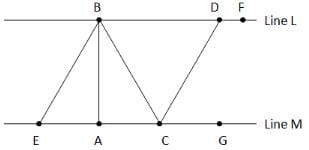If angle ABC is 30 degrees, what is the area of triangle BCE?
1)Angle CDF is 120 degrees, lines L and M are parallel, and AC = 6, BC = 12, and EC = 2AC
2)Angle DCG is 60 degrees, angle CDG is 30 degrees, angle FDG = 90, and GC = 6, CD = 12 and EC = 12

Detailed Solution for Test: Data Sufficiency- 4 - Question 8

Even though lines L and M look parallel and angle BAC looks like a right angle, you cannot make these assumptions.
The formula for the area of a triangle is .5bh
Evaluate Statement (1) alone.
Since EC = 2AC, EA = CA, EC = 2(6) = 12 and line AB is an angle bisector of angle EBC. This means that angle ABC = angle ABE. Since we know that angle ABC = 30, we know that angle ABE = 30. Further, since lines L and M are parallel, we know that line AB is perpendicular to line EC, meaning angle BAC is 90.
Since all the interior angles of a triangle must sum to 180:
angle ABC + angle BCA + angle BAC = 180
30 + angle BCA + 90 = 180
angle BCA = 60
Since all the interior angles of a triangle must sum to 180:
angle BCA + angle ABC + angle ABE + angle AEB = 180
60 + 30 + 30 + angle AEB = 180
angle AEB = 60
This means that triangle BCA is an equilateral triangle.
To find the area of triangle BCE, we need the base (= 12 from above) and the height, i.e., line AB. Since we know BC and AC and triangle ABC is a right triangle, we can use the Pythagorean theorem on triangle ABC to find the length of AB.
62 + (AB)2 = 122
AB2 = 144 - 36 = 108
AB = 1081/2
Area = .5bh
Area = .5(12)(1081/2) = 6*1081/2
Statement (1) is SUFFICIENT
Evaluate Statement (2) alone.
The sum of the interior angles of any triangle must be 180 degrees.
DCG + GDC + CGD = 180
60 + 30 + CGD = 180
CGD = 90
Triangle CGD is a right triangle.
Using the Pythagorean theorem, DG = 1081/2
(CG)2 + (DG)2 = (CD)2
62 + (DG)2 = 122
DG = 1081/2
At this point, it may be tempting to use DG = 1081/2 as the height of the triangle BCE, assuming that lines AB and DG are parallel and therefore AB = 1081/2 is the height of triangle BCE. However, we must show two things before we can use AB = 1081/2 as the height of triangle BCE: (1) lines L and M are parallel and (2) AB is the height of triangle BCE (i.e., angle BAC is 90 degrees).
Lines L and M must be parallel since angles FDG and CGD are equal and these two angles are alternate interior angles formed by cutting two lines with a transversal. If two alternate interior angles are equal, we know that the two lines that form the angles (lines L and M) when cut by a transversal (line DG) must be parallel.
Since lines L and M are parallel, DG = the height of triangle BCE = 1081/2. Note that it is not essential to know whether AB is the height of triangle BCE. It is sufficient to know that the height is 1081/2. To reiterate, we know that the height is 8 since the height of BCE is parallel to line DG, which is 1081/2.
Since we know both the height (1081/2) and the base (CE = 12) of triangle BCE, we know that the area is: .5*12*1081/2 = 6*1081/2
Statement (2) alone is SUFFICIENT.
Since Statement (1) alone is SUFFICIENT and Statement (2) alone is SUFFICIENT

Test: Data Sufficiency- 4 - Question 9

If both x and y are positive integers less than 100 and greater than 10, is the sum x + y a multiple of 11?
1)x -y is a multiple of 22
2)The tens digit and the units digit of x are the same; the tens digit and the units digit of y are the same

Detailed Solution for Test: Data Sufficiency- 4 - Question 9

If both x and y are multiples of 11, then both x + y and x - y will be multiples of 11. In other words, if two numbers have a common divisor, their sum and difference retain that divisor.
In case this is hard to conceptualize, consider the following examples:
42 - 18 {both numbers share a common factor of 6}
=(6*7) - (6*3)
=6(7 - 3)
=6(4)
=24 {which is a multiple of 6}

49 + 14 {both numbers share a common factor of 7}
=(7*7) + (7*2)
=7(7+2)
=7*9
=63 {which is a multiple of 7}
However, if x and y are not both multiples of 11, it is possible that x - y is a multiple of 11 while x + y is not a multiple of 11. For example:
68 - 46 = 22 but 68 + 46 = 114, which is not divisible by 11.
The reason x - y is a multiple of 11 but not x + y is that, in this case, x and y are not individually multiples of 11.
Evaluate Statement (1) alone.
Since x-y is a multiple of 22, x-y is a multiple of 11 and of 2 because 22=11*2
If both x and y are multiples of 11, the sum x + y will also be a multiple of 11. Consider the following examples:
44 - 22 = 22 {which is a multiple of 11 and of 22}
44 + 22 = 66 {which is a multiple of 11 and of 22}

88 - 66 = 22 {which is a multiple of 11 and of 22}
88 + 66 = 154 {which is a multiple of 11 and of 22}
However, if x and y are not individually divisible by 11, it is possible that x - y is a multiple of 22 (and 11) while x + y is not a multiple of 11. For example:
78 - 56 = 22 but 78 + 56 = 134 is not a multiple of 11.
Statement (1) alone is NOT SUFFICIENT.
Evaluate Statement (2) alone.
Since the tens digit and the units digit of x are the same, the range of possible values for x includes:
11, 22, 33, 44, 55, 66, 77, 88, 99
Since each of these values is a multiple of 11, x must be a multiple of 11.
Since the tens digit and the units digit of y are the same, the range of possible values for y includes:
11, 22, 33, 44, 55, 66, 77, 88, 99
Since each of these values is a multiple of 11, y must be a multiple of 11.
As demonstrated above, if both x and y are a multiple of 11, we know that both x + y and x - y will be a multiple of 11.
Statement (2) alone is SUFFICIENT.
Since Statement (1) alone is NOT SUFFICIENT and Statement (2) alone is SUFFICIENT.

Test: Data Sufficiency- 4 - Question 10

If x and y are positive integers, is the following cube root an integer?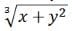1)x = y2(y-1)
2)x = 2

Detailed Solution for Test: Data Sufficiency- 4 - Question 10

Evaluate Statement (1) alone.
Substitute the value of x from Statement (1) into the equation and manipulate it algebraically.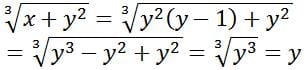Since the question says that y is a positive integer, you know that the cube root of y3, which equals y, will also be a positive integer. Statement (1) is SUFFICIENT.
Evaluate Statement (1) alone (Alternative Method).
For the cube root of a number to be an integer, that number must be an integer cubed. Consequently, the simplified version of this question is: "is x + y2 equal to an integer cubed?"
Statement (1) can be re-arranged as follows:
x = y3 - y2
y3 = x + y2
Since y is an integer, the cube root of y3, which equals y, will also be an integer.
Since y3 = x + y2, the cube root of x + y2 will also be an integer. Therefore, the following will always be an integer: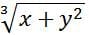Statement (1) alone is SUFFICIENT.
Evaluate Statement (2) alone.
Statement (2) provides minimal information. The question can be written as: "is the following cube root an integer?"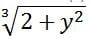If y = 4, x + y2 = 2 + 42 = 18 and the cube root of 18 is not an integer. However, if y = 5, x + y2 = 2 + 52 = 27 and the cube root of 27 is an integer. Statement (2) is NOT SUFFICIENT.
Since Statement (1) alone is SUFFICIENT and Statement (2) alone is NOT SUFFICIENT

## Quantitative for GMAT

72 videos|87 docs|154 tests
 Use Code STAYHOME200 and get INR 200 additional OFF Use Coupon Code
Information about Test: Data Sufficiency- 4 Page
In this test you can find the Exam questions for Test: Data Sufficiency- 4 solved & explained in the simplest way possible. Besides giving Questions and answers for Test: Data Sufficiency- 4, EduRev gives you an ample number of Online tests for practice

## Quantitative for GMAT

72 videos|87 docs|154 tests